# Complex Conjugate| Modulus of Complex numbers

## Conjugate of Complex Numbers

Let z be the complex number defined as
$z=x+iy$
The conjugate of z is defined as
$\bar{z}=x-iy$
So by defination of Conjugate of any complex number is obtained by replacing i with -i

## Properties of Conjugate Number

For $z=x+iy$
1. $\bar(\bar{z})=z$
Proof
$z=x+iy$
$\bar{z}=x-iy$
$\bar(\bar{z}) =x+iy =z$

2. $z+\bar{z}=2x$
Proof
$z=x+iy$
$\bar{z}=x-iy$
$z+\bar{z} =x+iy +x-iy=2x$

3. $z-\bar{z}=2iy$
Proof
$z=x+iy$
$\bar{z}=x-iy$
$z -\bar{z} =x+iy -(x-iy)=2iy$

4. if $\bar{z}=z$ then it means z is real number
Proof
$z=x+iy$
$\bar{z}=x-iy$
$\bar{z}=z$
x+iy=x-iy
or y=0
So z=x ,So it is a real number

5. if $z+\bar{z}=0$ then it means z is pure imaginary number
Proof
$z=x+iy$
$\bar{z}=x-iy$
$z+\bar{z}=0$
x+iy+x-iy=0
or x=0

So z=iy ,So it is a pure imaginary number

6. $z\bar{z}=(x^{2}+y^{2})$
Proof
$z=x+iy$
$\bar{z}=x-iy$
$z\bar{z}=(x+iy)(x-iy) = (x^{2}-i^{2}y^{2}) =(x^{2}+y^{2})$

7.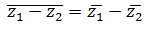Proof
Let z1 = a + ib and z2 = c + id
then $\bar{z_1} = a - ib$ and $\bar{z_2} = c - id$
Now, $\bar{z_1 - z_2} = \bar{a + ib - c -id} = \bar{a - c +i(b - d)}=a + c - i(b - d)$

Also $\bar{z_1} - \bar{z_2} =a - ib - c + id=a - c - i(b - d)$
Hence Proved

8.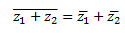Proof
Let z1 = a + ib and z2 = c + id
then $\bar{z_1} = a - ib$ and $\bar{z_2} = c - id$
Now, $\bar{z_1 + z_2} = \bar{a + ib + c + id} = \bar{a + c + i(b + d)}=a + c - i(b + d)$

Also $\bar{z_1} + \bar{z_2} =a - ib +c - id=a + c - i(b + d)$
Hence Proved

9.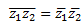Proof
Let z1 = a + ib and z2 = c + id
then $\bar{z_1} = a - ib$ and $\bar{z_2} = c - id$
Now, $\bar{z_1 z_2} = \bar{ac - bd + i(ad + bc)} = ac - bd + i(ad + bc)$

Also $\bar{z_1} \bar{z_2} =ac - bd + i(ad + bc)$
Hence Proved

10.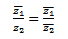when $z_{2}$ is not zero
Proof
Let z1/z2 = z3
then z1=z2z3
$\bar{z_1}=\bar{z_2 z_3}= \bar{z_2}\bar{z_3}$
or $\bar{z_3}= \frac {\bar{z_1}} {\bar{z_2}}$ or $\bar {\frac {z_1}{z_2}} =\frac {\bar{z_1}} {\bar{z_2}}$
Hence Proved

Example:
z=2-3i
Then
$\bar{z}=2+3i$

## Modulus Of complex Number

The module of a complex number
$z=x+iy$
is defined as |z|
|z|=$\sqrt{(x^2+y^2)}$
Clearly |z| >= 0

## Properties of Modulus of Complex Number

For $z=x+iy$
1. |z| =0 then it mean x=y=0
2. $|z|=|\bar{z}|=|-z|$
3. $z\bar{z}=|z|^{2}$
Proof
Let z = a + ib, then $\bar{z} = a - ib$
Now LHS
$z\bar{z} = (a + ib)(a - ib)=a^2 -i^2b^2 =a^2+b^2$
RHS
$|z|^{2}= a^2+b^2$
Hence Proved
4. $|z_{1}z_{2}|=|z_{1}||z_{2}|$
5.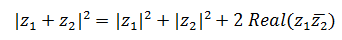6.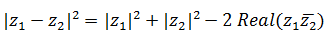7. $|\frac{z_{1}}{z_{2}}|=\frac{|z_{1}|}{|z_{2}|}$ when $z_{2}$ is not zero
8. The proof of the above properties are quite self explanatory
Example
z=3-4i
Then
|z|=5

## Reciprocal or Multiplicative Inverse of Complex Number using Complex Conjugate

z= x+iy
Then
$\frac{1}{z}=\frac{\bar{z}}{|z|^{2}}$

Examples
(1) (3 + i)(1 + 7i)
Solution:
(3 + i)(1 + 7i)= 3×1 + 3×7i + i×1+ i×7i
= 3 + 21i + i + 7i2
= 3 + 21i + i - 7 (because i2 = -1)
= -11 + 22i
We can generalized this multiplication
(a+bi)(x+ui) = (ax-by) + (ay+bx)i

(2) Solving the equation
(1+2i)z=(1-i)
Solution:
$z=\frac{1-i}{1+2i}$
Dividing the conjugate for denominator
$z=[\frac{1-i}{1+2i}][\frac{1-2i}{|1-2i}]$
$z=[\frac{1-2i-i+2}{5}]$
$z=[\frac{3-i}{5}]$

### Quiz Time

Question 1
The conjugate of the complex number $\frac {1-i}{1+i}$ is
A) $-i$
B) $i$
C) ${1-i}$
D) ${1+i}$
Question 2
The conjugate of the complex number $1 + i^{-35}$? A) $1 - i$
B) $1 +i$
C) $i$
D) None of the above
Question 3
Find the value $|(3 +4i)(1-i)|$
A) $5 \sqrt{2}$
B) $5 \sqrt{3}$
C) $\sqrt{2}$
D)none of the above
Question 4
which of following is incorrect for complex number $z=a+ib$ and its conjugate $\bar{z}$
A) $z \bar{z}= a^2 + b^2$
B) $z + \bar{z} = 2a$
C) $\bar{z} -z = 2bi$
D) $\bar(\bar{z})=z$
Question 5
if $z_1= 5+ i \sqrt{2}$ and $z_2= 1-i \sqrt {2}$,then $\frac {z_1}{z_2}$ is equal to
A) $1 - i$
B) $1 + i$
C)$1 -2 \sqrt {2} i$
D)$1 +2 \sqrt {2} i$
Question 6>which is of these is incorrect
A)$|z_{1} + z_{2}| \neq |z_{1}|+ |z_{2}|$
B) $|z_{1} - z_{2}| \geq |z_{1}| - |z_{2}|$
C) $|z| \geq |-z|$
D) $|z_{1} z_{2}| = |z_{1}||z_{2}|$

Go back to Class 11 Main Page using below links

Note to our visitors :-

Thanks for visiting our website.